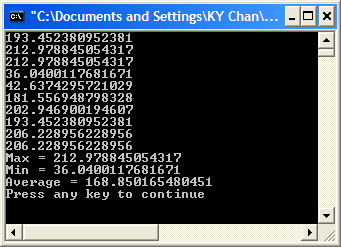The following information is for the EMANT300, EMANT380

# Exercise 11 – Array and Basic Statistics

## Objective

• Learn about Arrays

• Do Basic Statistics

Sam has been asked to help out in a Greenhouse project. He was told that light intensity and duration are important for crop growth and development as photosynthesis uses light. He has the following information

• Low light causes plants to be long and spindly, have small leaves, bud blades, poor pollination and poor fruit quality.

• Photosynthesis is stopped at high light intensity depending on species.

Sam decided that on top of taking light intensity measurements, he would like to know some basic statistics of the measurements like the maximum, minimum and average light intensities. One way to do this is to use arrays.

1.Open the Visual Basic solution VB_2010_Solution.sln.

2. Set the LightArray project as the startup project.

3. View the project code by opening the project's Module1.vb

4.If you are using the EMANT380 Bluetooth DAQ, change the parameter to False and COM5 to the COM port you are using, See exercise 1 step 4.

``DAQ.Open(False,"COM5")``

5. Press Ctrl+F5 to Start without Debugging to run the program. 10 Light Intensity measurements in Lux will be displayed at the rate of 1 measurement per second. At the end of the measurement, basic statistics like maximum, minimum and average light intensities will be displayed.6. Press any key to return to the development environment.

## Program 11.1 Array and Basic Statistics

``````Imports Emant
Module Module1
Sub Main()
Dim i As Integer
Dim volt, lux As Double
Dim luxarray(9) As Double
Dim DAQ As Emant300 = New Emant300
DAQ.Open(True, "COM5")
For  i = 0 To 9
volt = DAQ.ReadAnalog(Emant300.AIN.AIN0, Emant300.AIN.COM)
lux = 1333 * volt
Console.WriteLine(lux)
luxarray(i) = lux
DAQ.Delay(1000)
Next
Console.WriteLine("Max  = {0}", luxarray.Max)
Console.WriteLine("Min  = {0}", luxarray.Min)
Console.WriteLine("Ave  = {0}", luxarray.Average)
DAQ.Close()
End Sub
End Module``````If you are writing this program from scratch, in order to use the Emant300 class, the class library Emant300.dll must be added to the references folder. See exercise 5.

## Array

``Dim luxarray(9) As Double``

An array is a data structure that contains a number of variables which are accessed through computed indices.

``luxarray(i) = lux``

The variables contained in an array, also called the elements of the array, are all of the same type, and this type is called the element type of the array. luxarray elements are of the double data type

An array has a rank which determines the number of indices associated with each array element. The rank of an array is also referred to as the dimensions of the array. An array with a rank of one is called a single-dimensional array. An array with a rank greater than one is called a multi-dimensional array. Specific sized multi-dimensional arrays are often referred to as two-dimensional arrays, three-dimensional arrays, and so on. luxarray is a one dimensional array

Each dimension of an array has an associated length which is an integral number greater than or equal to zero. The dimension lengths are not part of the type of the array, but rather are established when an instance of the array type is created at run-time. The length of a dimension determines the valid range of indices for that dimension. The index upper bound in the array variable declaration determines the length of the array. luxarray array length is 10.

For a dimension of length N, indices can range from 0 to N – 1 inclusive. The total number of elements in an array is the product of the lengths of each dimension in the array. If one or more of the dimensions of an array have a length of zero, the array is said to be empty.

The element type of an array can be any type, including an array type.

## Statistics

``````    Console.WriteLine("Max  = {0}", luxarray.Max)
Console.WriteLine("Min  = {0}", luxarray.Min)
Console.WriteLine("Ave  = {0}", luxarray.Average)``````

An aggregation operation computes a single value from a collection of values. An example of an aggregation operation is calculating the average light intensity from 10 lux values.

## Console Formatting

``Console.WriteLine("Max = {0}", luxarray.Max)``

The console replaces {0} with the result of the method luxarray.Max More information on the formatting can be found from the Microsoft website.